Nie powiodło nam się wysłanie linku mailem. Prosimy o sprawdzenie Państwa poczty email.

# Pomoc Online

Tree
Settings
Produkt:
Program:
Język:

## Influence of Water

Ground water can be assigned to the slope plane section using one of the five options:

## 1) Ground water table

The ground water table is specified as a polygon. It can be arbitrarily curved, placed totally within the soil body or introduced partially above the ground surface.

Presence of water influences value of pore pressure acting within a soil and reducing its shear bearing capacity. The pore pressure is considered as the hydrostatic pressure, i.e. unit weight of water is multiplied by reduced height of the water table: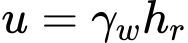where: γw - unit weight of water hr - reduced height of water table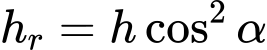where: h - vertical distance of point, where pore pressure is calculated and point on the water table α - inclination of the water table

Resultant force of pore pressure at certain section of the block is used in the calculation: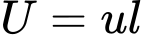where: u - pore pressure in the point l - length of section

Below the ground water table the analysis proceeds using the unit weight of saturated soil γsat and uplift pressure; above the ground water table the analysis assumes the input unit weight of soil γ.

The shear forces along the slip surface are provided by: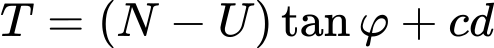where: T - shear force along slip surface segment N - normal force along slip surface segment U - pore pressure resultant along slip surface segment φ - angle of internal friction c - cohesion d - length of slip surface segment

In case of total stress (entered in the "Soil" dialog window) total parameters are used and pore pressure is considered zero.

## 2) Ground water table including suction

Suction table can be introduced above the input ground water table. A negative value of pore pressure u is then assumed with the region separated by the two tables. Suction increases as negative hydrostatic pressure from the ground water table towards the suction table.

## 3) Rapid draw down

Original table can be introduced above the input ground water table. Original water table simulates state before rapid drawdown.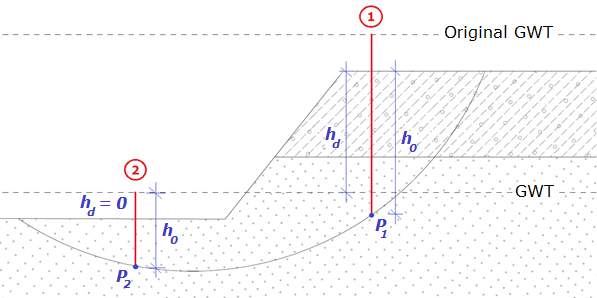Rapid draw down analysis

First of all, the initial pore pressure u0 is evaluated: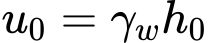where: h0 - height from original table to the point of evaluation P γw - unit weight of water

Height h0 is generally the distance from point of pore pressure evaluation (P) to original water table - this is valid for the case when original water table is under terrain surface. In case of original water table above terrain there is used the height h0 from point P to the level of terrain surface (profile 1 in the figure). Another case is when original water table as well as ground water table are both above terrain - then height h0 is the distance from ground water table to point P (profile 2 in the figure).

Second step is to calculate change of pore pressure in the area between original and ground water table: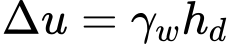where: hd - height from original to ground water table γw - unit weight of water

As in previous calculation of pressure, there are three possibilities how to get height hd. When both water tables are under terrain, hdis the distance between original and ground water table. In case of original water table above terrain, then hd is measured from ground water table to the level of terrain (profile 1 in the figure). Last case is when both water tables are above terrain - then height hd is zero (profile 2 in the figure).

Third step is calculation of final value of pore pressure u. Change of pore pressure Δu is multiplied by coefficient of reduction of initial pore pressure X, which is required for all soils (dialog window "Soils").  X coefficient of the soil in the area of point P is used (NOT soil in the area between original and ground water table). In case of permeable soil X = 1, in other case X = 0. Final pore pressure is evaluated as: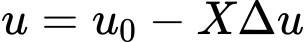where: u0 - initial pore pressure X - coefficient of reduction of initial pore pressure Δu - change of pore pressure

## 4) Coefficient of pore pressure Ru

The coefficient of pore pressure Ru represents the ratio between the pore pressure and geostatic pressure in a soil body. In the area, where Ru is positive, entered unit weight of saturated soil γsat is considered; in other case unit weight of soil γ is used.

The values of Ru are introduced with the help of isolines connecting points with the same value of Ru. Linear interpolation is assumed to obtain intermediate values. Pore pressure is established as geostatic stress reduced by coefficient Ru:where: Ru - coefficient of pore pressure hi - height of ith soil layer γi - unit weight of ith soil layer

## 5) Pore pressure values

Ground water can be introduced directly through the pore pressure values u within the plane section of a soil body.

In the area, where u is positive, entered unit weight of saturated soil γsat is considered; in other case unit weight of soil γ is used.

The pore pressure values are introduced with the help of isolines connecting points with the same value of pore pressure. Linear interpolation is assumed to obtain intermediate values. Pore pressure values are then derived from the values of pore pressure obtained in specific points within the slope plane section.